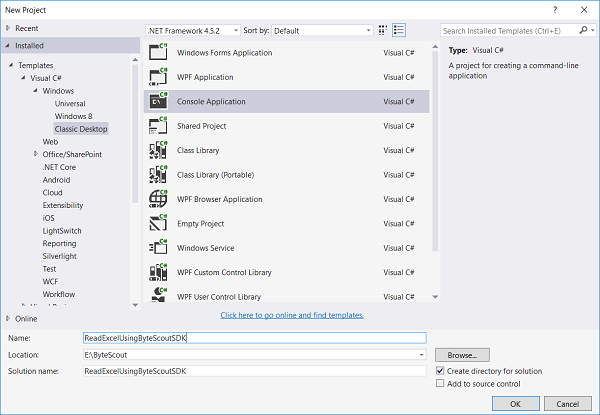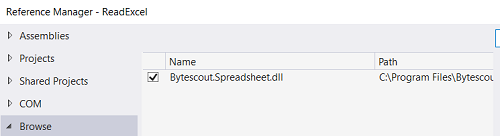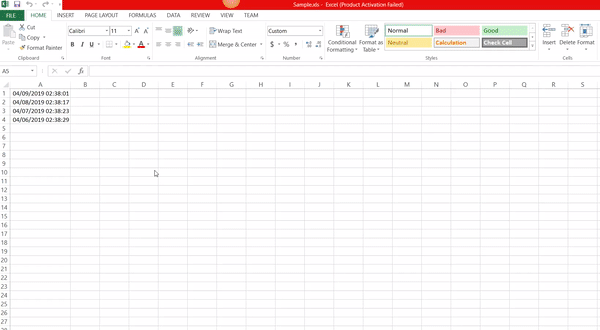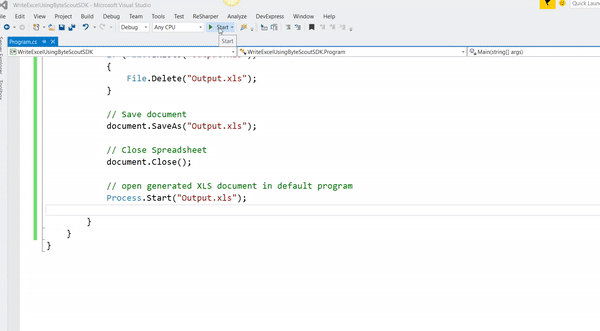Read and Write Excel Files in C# - Source Code - Read From Excel File

# Read and Write Excel files in C# – Sample Source Codes – Read from Excel File

• Home
• /
• Articles
• /
• Read and Write Excel files in C# – Sample Source Codes – Read from Excel File

Read and Write Excel files in C# tutorial shows how to write to and read from Excel file from your application using Speadsheet SDK. Use sample C# source codes for Excel reading and writing. Get the most of this tutorial by learning the source samples. Read the Excel file in C# with the help of the code below.

## Write to Excel file from your application

```using System;
using System.Collections.Generic;
using System.Text;
using System.Diagnostics;
using System.IO;

namespace HelloWorld
{
class Program
{
static void Main(string[] args)
{

// headers to indicate purpose of the column
Sheet.Cell("A1").Value = "Formula (as text)";
// set A column width
Sheet.Columns.Width = 250;

Sheet.Cell("B1").Value = "Formula (calculated)";
// set B column width
Sheet.Columns.Width = 250;

// write formula as text
Sheet.Cell("A2").Value = "7*3+2";
// write formula as formula
Sheet.Cell("B2").Value = "=7*3+2";

// delete output file if exists already
if (File.Exists("Output.xls")){
File.Delete("Output.xls");
}

// Save document
document.SaveAs("Output.xls");

document.Close();

// open generated XLS document in default program
Process.Start("Output.xls");

}

}
}```

## How to read excel file in C# console application:

```using System;
using System.Collections.Generic;
using System.Text;
using System.Diagnostics;
using System.IO;

namespace HelloWorld
{
class Program
{
static void Main(string[] args)
{

// Get worksheet by name
Worksheet worksheet = document.Workbook.Worksheets.ByName("Sheet1");

// Check dates
for (int i = 0; i &amp;amp;amp;amp;amp;lt; 4; i++)
{
// Set current cell
Cell currentCell = worksheet.Cell(i, 0);

DateTime date = currentCell.ValueAsDateTime;

// Write Date
Console.WriteLine("{0}", date.ToShortDateString());
}

// Close document
document.Close();

// Write message
Console.Write("Press any key to continue...");

// Wait user input

}
}
}```

Microsoft Excel is such a powerful software with a lot of features and is mostly used for data analysis. Many times your customers ask you to read or write into an Excel file. In earlier days we generally used to do that using an interop object, but it is unmanaged and kills your application performance.

ByteScout Spreadsheet SDK is such an efficient library that uses its own custom engine to easily read or write Excel files. Before, manipulating Microsoft Excel files in web or windows applications was really a tedious task. But ByteScout Spreadsheet SDK makes our life much easier. It simplifies the process of reading and writing to excel files and hides the complexities involved without needing to deal with Interop. Even no Excel installation is required when we need to write or read into Excel files using this library.

So who are we waiting for? Let’s get started:

## * Steps to read data from an Excel file using c#:

### Step 1: Create a new C# console application project.### Step 3: Include the following namespaces in the Program.cs file.

`using System;`

`using Bytescout.Spreadsheet;`

### Step 4: Add the following code snippet to read data from an Excel file.

```                class Program
{
static void Main(string[] args)
{

// Get worksheet by name
Worksheet worksheet = document.Workbook.Worksheets.ByName("Sheet1");

// Check dates
for (int i = 0; i < 4; i++)
{
// Set current cell
Cell currentCell = worksheet.Cell(i, 0);

DateTime date = currentCell.ValueAsDateTime;

// Write Date
Console.WriteLine("{0}", date.ToShortDateString());
}

// Close document
document.Close();

// Write message
Console.Write("Press any key to continue...");

// Wait user input

}
}
```

And here’s the output.I think no need to explain the above code as we simply create an instance of Spreadsheet object and load the physical excel file(resides in bin\Debug folder) into it and read the content of the worksheet by specifying its name. Well, the above sample code is in c#, but you can easily achieve the same thing in VB.Net also.

## * Steps to write data into an Excel file using c#:

Let’s see this step-by-step.

### Step 1: Create a new C# console application project.### Step 3: Include the following namespaces in the Program.cs file.

`using Bytescout.Spreadsheet;`
`using System.Diagnostics;`
`using System.IO;`

```
class Program
{
static void Main(string[] args)
{

// headers to indicate purpose of the column
Sheet.Cell("A1").Value = "Formula (as text)";
// set A column width
Sheet.Columns.Width = 250;

Sheet.Cell("B1").Value = "Formula (calculated)";
// set B column width
Sheet.Columns.Width = 250;

// write formula as text
Sheet.Cell("A2").Value = "7*3+2";
// write formula as formula
Sheet.Cell("B2").Value = "=7*3+2";

// delete output file if exists already
if (File.Exists("Output.xls")){
File.Delete("Output.xls");
}

// Save document
document.SaveAs("Output.xls");

document.Close();

// open generated XLS document in default program
Process.Start("Output.xls");
}
}
```

The output is as follows.Let’s understand the above code step by step.

First, we create an instance of Spreadsheet object.

`            // Create new Spreadsheet`

`            Spreadsheet document = new Spreadsheet();`

And then we add the new worksheet in a newly created object of Spreadsheet using the following code.

`             // add new worksheet`

`            Worksheet Sheet = document.Workbook.Worksheets.Add("FormulaDemo");`

• Workbook – Represents a single workbook within the Spreadsheet object
• Worksheet – A member of the Worksheets collection in the Workbook object

And then we set a two-column header name and its width and then write the formula in the respected columns.

```               // headers to indicate purpose of the column
Sheet.Cell("A1").Value = "Formula (as text)";
// set A column width
Sheet.Columns.Width = 250;

Sheet.Cell("B1").Value = "Formula (calculated)";
// set B column width
Sheet.Columns.Width = 250;

// write formula as text
Sheet.Cell("A2").Value = "7*3+2";
// write formula as formula
Sheet.Cell("B2").Value = "=7*3+2";
```
• And then we check if the same file name already exists in the application’s `bin\Debug folder`. If exists then first delete it.

`               // delete output file if exists already`

`               if (File.Exists("Output.xls")){`

`                        File.Delete("Output.xls");`

`                }`

• And then we save the newly generated file.

`               // Save document`

`               document.SaveAs("Output.xls");`

In this article, we provided a solution on how to read an Excel file and write into an Excel file using ByteScout Spreadsheet SDK in C#. For that, we have created two sample console applications and write the code step-by-step. The best way to evaluate the SDK and get to know the code is to debug it and make small changes to see what happens 🙂